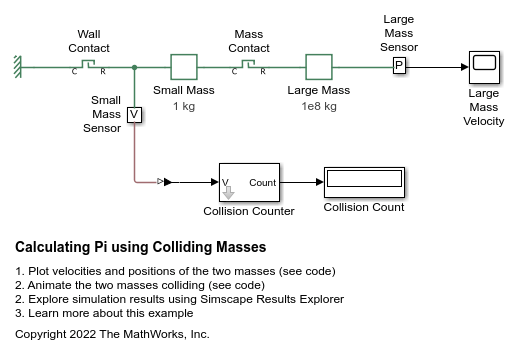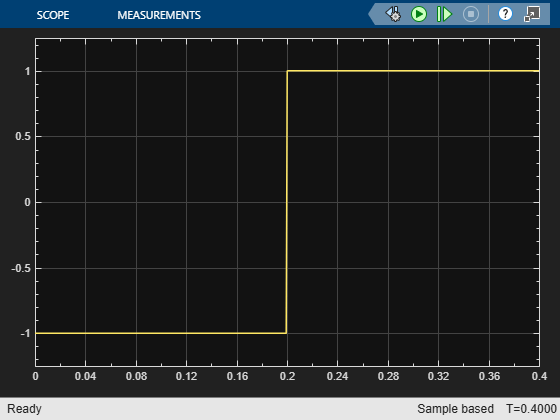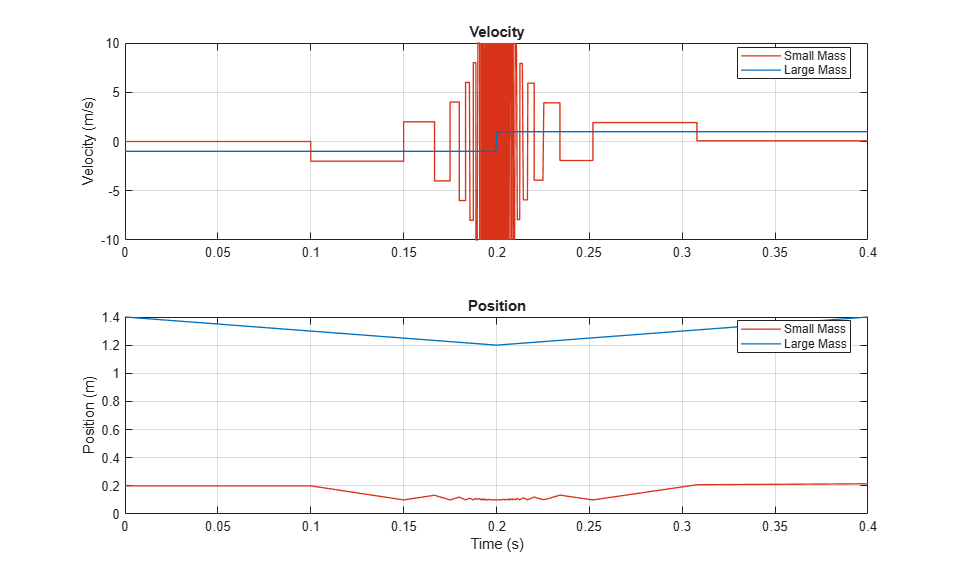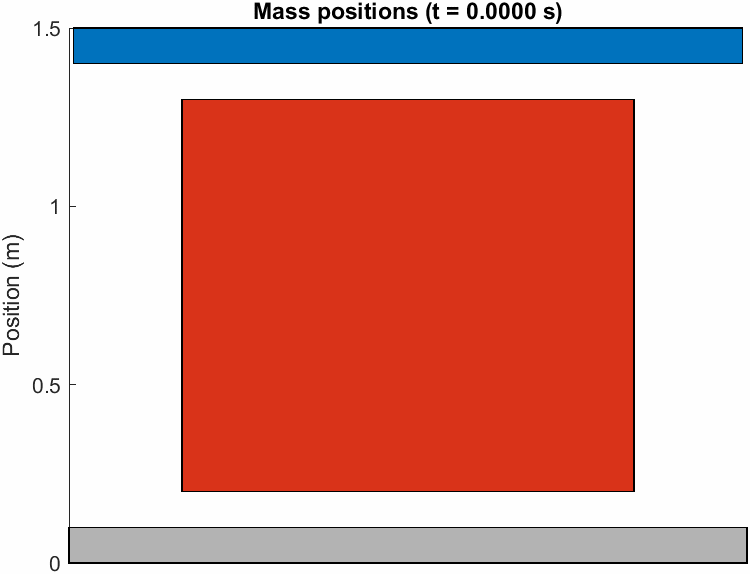# Calculating Pi Using Colliding Masses

This example uses a well-known physics problem to demonstrate solver performance by capturing tens of thousands of instantaneous events that occur in under a second. On a one-dimensional path, a large mass approaches a small mass bounded by a wall on the far side. As the large mass strikes the small mass, the small mass bounces off the wall and reverses direction toward the large mass. Each collision is perfectly elastic. As the large mass approaches the wall, collisions with the small mass occur more and more rapidly until the large mass reverses direction and eventually travels fast enough in the opposite direction that the small mass never catches it.

When the large mass in 100^n times larger than the small mass, the exact number of total collisions is the first n+1 digits of pi. This result occurs because of the relationship between the conservation of energy and the conservation of momentum. If you plot the square roots of kinetic energies of the two masses on orthogonal axes, the system always exists on a point along a circumference whose radius depends on the total energy of two masses. Each collision moves the system to a new point on the circumference from one side to the other. Collisions with the wall cause the point to move vertically. Collisions with the large mass cause the point to move at a slope equal to the negative square root of the mass ratio.

The model uses Hard Stop blocks with the coefficient of restitution setting to represent the elastic collisions. The solver captures a total of 31415 collisions in 0.4 seconds. The collision counter block is a custom block designed to capture the collision events.

### Model### Simulation Results from Scopes### Simulation Results from Simscape Logging

This figure plots the positions and velocities of the 2 masses. It shows the instantaneous changes in velocity each time the small mass collides with the wall or the large mass and the change in velocity of the large mass when the small mass reverses the large mass's direction.### Animation of Simscape Logging Results

This figure shows the positions of the colliding masses over time. The red block in the middle represents the small mass, the blue block at the top represents the large mass, and the gray block at the bottom represents the wall.